# Likelihood method for estimating electron charge mis-identification rates

Dear Rootians,

I am interested in computing the charge miss identification rates. These rates are derived from the data, based on the fraction of Z ->ee. The events in the m_ee region around the reconstructed Z-boson peak mZ are used.

For Nij electron pairs falling in the bin combination i,j the expected number of same-sign events is: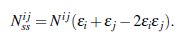where ϵi and ϵj are the QMisID rates for each of the two electrons.

If all the same-sign events in the Z peak are produced by charge flip, then these numbers are described by a Poisson distribution: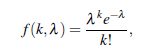Where observed events =>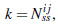and expected events =>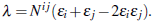The probability for both electrons to produce a charge flip is: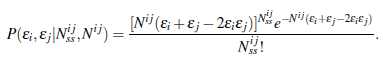Which is integrated into likelihood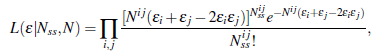that can be maximised (minimisation of -2lnL) to obtain the rates that best describe the data.

So for that, I have draw the distribution of Z->ee and apply the fit using the likelihood fit.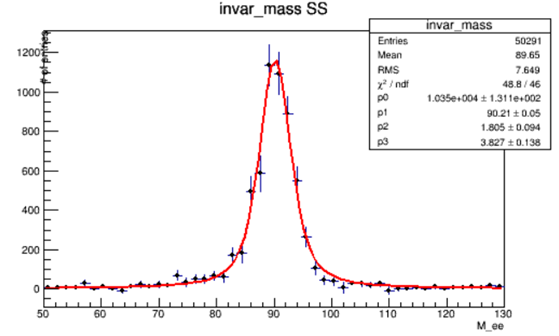the fit results are as follows

``````****************************************
MinFCN                    =      272.163
Chi2                      =      48.8082
NDf                       =           46
Edm                       = 7.15068e-008
NCalls                    =           91
p0                        =        10345   +/-   425.092
p1                        =       90.205   +/-   0.162891
p2                        =      1.80446   +/-   0.374657
p3                        =      3.82712   +/-   0.528939
``````

What I did not understand is that …what will be the QmisID rates( ϵi,ϵj) form the likelihood fit results?
Many thanks@moneta Can you comment?

1 Like

Hi,
In the fit above, which function did you use for fitting ? How did you code it ?
The parameters p0,…p4 are the parameters in teh order you have defined in your fit model function.

Lorenzo

Hi @moneta ,
For fitting, I have tried different functions like Gaus, Lorentzian, Double sided CB and Voigt function etc.

Base on good Chi2 value, for the above fit, I am using Voigt (convolution of gauss and lorentz ) function.
The code that I am using for fit is here.

``````#include "TFile.h"
#include "TCanvas.h"
#include "TTree.h"
#include "TH1F.h"
#include "TF1.h"
#include "TMinuit.h"
#include "TMath.h"

Double_t funVoigt(Double_t *x, Double_t *par) {
return (par * TMath::Voigt(x - par, par, par, 4)) ;
}

int Voigt(){

TFile *file = new TFile( "Zjets_SS.root" );
TH1F * h_mee = (TH1F*)file->Get("invar_mass");

TF1 *f1 = new TF1("f1","funVoigt",50,130,4);

double par;
par=10000;
par=90;
par=2;
par=3;
f1->SetParameters(&par);

TCanvas *C = new TCanvas("C","C",800,600);
TFitResultPtr r = h_mee->Fit(f1,"SWLR", "ep");
TMatrixDSym cov = r->GetCovarianceMatrix();  //  to access the covariance matrix
Double_t chi2   = r->Chi2(); // to retrieve the fit chi2
Double_t par0   = r->Parameter(0); // retrieve the value for the parameter 0
Double_t err0   = r->ParError(0); // retrieve the error for the parameter 0
r->Print("V");     // print full information of fit including covariance matrix
gStyle->SetOptFit(111);

return 0;
}
``````

Hi,

Then it is clear what the parameters p[] are. I guess your parameters can be derived by the number of expected Z->ee events. This can be obtained from the integral of your fitted function, assuming you don’t have a background component in your data.

Lorenzo

This topic was automatically closed 14 days after the last reply. New replies are no longer allowed.